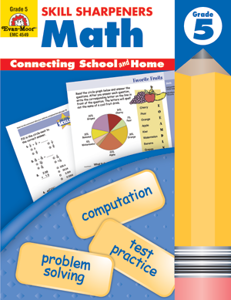# Skill Sharpeners Math, Grade 5

EMC4549
Regular price \$10.99 \$0.00

Skill Sharpeners Math, Grade 5 provides practice for the following mathematics skills:

• division with remainders
• solving multiple-step word problems
• multiplying decimals and fractions
• identifying factors
• prime and composite numbers
• calculating perimeter and area
• interpreting graphs
• solving logic puzzles
• identifying probabilities
• range, mean, median, and mode
• measuring angles
• calculating equivalent fractions, decimals, and percents